# GMAT Math : Calculating whether point is on a line with an equation

## Example Questions

### Example Question #1 : Calculating Whether Point Is On A Line With An Equation

Solve forin the coordinate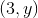on line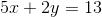?Explanation:

To solve forfor, we have to pluginto thevariable of the equation and solve for: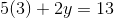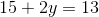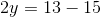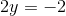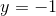### Example Question #901 : Gmat Quantitative Reasoning

Solve forin the coordinate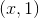on line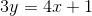?Explanation:

To solve forfor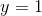, we have to plug 1 into thevariable of the equation and solve for: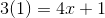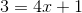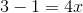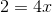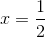### Example Question #71 : Coordinate Geometry

Solve forin the coordinate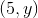on line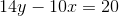?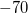Explanation:

To solve forfor, we have to pluginto thevariable of the equation and solve for: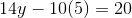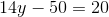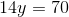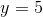### Example Question #1 : Calculating Whether Point Is On A Line With An Equation

Consider segment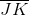which passes through the points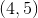and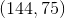.

If the point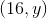is on, what is the value of y?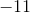Explanation:

First, use the points to find the equation of JK:

Given that JK passes through (4,5) and (144,75) we can find the slope as follows:

Slope is found via: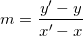Plug in and calculate: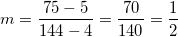Next, we need to use one of our points and the slope to find our y-intercept. I'll use (4,5).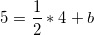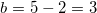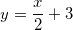To find y, we need to plug in 16 for x and solve: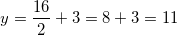### Example Question #73 : Coordinate Geometry

Ifis defined as follows, is the point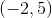on?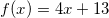No

Cannot be calculated from the provided information

Yes

f(x) is undefined at (-2,5)

Yes

Explanation:

To find out if (-2,5) is on f(x), simply plug the point into f(x)Becomes,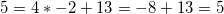So yes, it does!

### Example Question #911 : Gmat Quantitative Reasoning

Which of the following are points along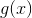if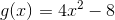.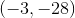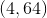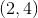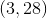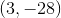Explanation:

One way to solve this one is by plugging in each of the answer choices and eliminating any that don't work out. Begin with our original g(x)If we plug in 3 we get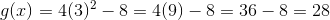So our point is (3,28).

### All GMAT Math Resources